### Choose language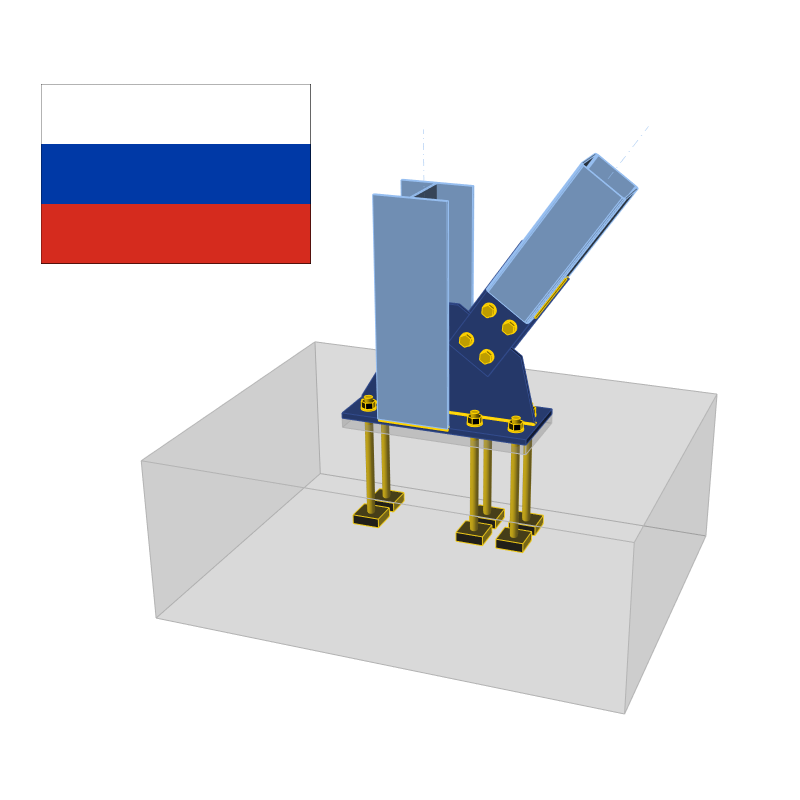# Check of components according to SP (Russian standards)

$$CBFEM method combines advantages of the general Finite Element Method (FEM) and standard Component Method (CM). The stresses and internal forces calculated on the accurate CBFEM model are used in checks of all components – Bolts, preloaded bolts, and welds which are checked according to SP 16.13330.2017. The concrete in bearing is checked according to SP 63.13330.2012. The plates are checked by finite element analysis. The checks of anchorage have not yet been implemented in the current version. Design check of plates according to SP Strain check is performed at shell finite elements simulating plates. The yield strength is divided by factor for material strength and multiplied by the service factor.The resulting equivalent stress (HMH, von Mises) and plastic strain are calculated on plates. When the yield strength (divided by partial safety factor for material strength, γm – SP 16, Table 3, and multiplied by service factor γc – SP 16, Table 1, which is editable in Code setup, SP 16, Cl. 11.1.1) on the bilinear material diagram is reached, the check of the equivalent plastic strain is performed. The limit value of 5 % is suggested in Eurocode (EN 1993-1-5 App. C, Par. C8, Note 1). This value can be modified in Code setup but verification studies were made for this recommended value. The material properties of the member is determined by the thickest plate.$\frac{1}{R_y \gamma_c} \sqrt{\sigma_x^2-\sigma_x \sigma_y + \sigma_y^2 + 3 \tau_{xy}^2} \le 1.0$Plate element is divided into 5 layers and elastic/plastic behaviour is investigated in each of them. The program shows the worst result from all of them.Stress may be a little bit higher than design yield strength. The reason is the slight inclination of the plastic branch of the stress-strain diagram, which is used in the analysis to improve the stability of the calculation. Check of bolts and preloaded bolts according to SP BoltsBolts are checked according to SP 16, Cl. 14.2. The tensile and shear force in each bolt is determined by finite element analysis. Prying forces are determined by finite element analysis and taken into account. Each shear plane is checked individually. Ply in bearing is checked against the sum of shear forces at nearby planes.Bolt in shearA bolt subject to a design shear force is designed according to Cl. 14.2.9 and shall satisfy:$N_s \le N_{bs} = R_{bs} A_b \gamma_b \gamma_c$where: Ns – shear force in one plane of a bolt Nbs – bolt shear resistance Rbs – design shear strength of a bolt – SP 16, Table 5 Ab – bolt gross section area γb – service factor of bolt joint – SP 16, Table 41 – γb = 1.0 for single bolting and multibolting with accuracy class A, γb = 0.9 for multibolting and accuracy class B and high strength bolts (Rbun ≥ 800 MPa) γc – service factor – SP 16, Table 1, editable in Code setup Rbyn [MPa]Rbs [MPa] $$R_{byn} \le 300$$$$0.42 \cdot R_{bun}$$ $$300 < R_{byn} \le 400$$$$0.41 \cdot R_{bun}$$ $$400 < R_{byn} \le 936$$$$0.40 \cdot R_{bun}$$ $$936 > R_{byn}$$$$0.35 \cdot R_{bun}$$ Each shear plane is checked individually.Bolt in tensionA bolt subject to design tension force is designed according to SP 16, Cl. 14.2.9 and shall satisfy:$N_t ≤ N_{bt} = R_{bt} A_{bn} \gamma_c$where: Nt – tensile force in a bolt Nbt – bolt tensile resistance Rbt – design tensile strength – SP 16, Table 5 Abn – net cross-sectional area of a bolt γc – service factor – SP 16, Table 1, editable in Code setup Rbun [MPa]Rbt [MPa] $$R_{bun} < 830$$$$0.45 \cdot R_{bun}$$ $$830 \le R_{bun} < 1040$$$$0.54 \cdot R_{bun}$$ $$R_{bun} \ge 1040$$$$0.70 \cdot R_{bun}$$ Bolt subject to combined shear and tensionA bolt loaded in shear and tensile forces at the same time is designed according to SP 16, Cl. 14.2.13 and shall satisfy:$\sqrt{\left ( \frac{N_t}{N_{bt}} \right ) ^2 + \left ( \frac{N_s}{N_{bs}} \right ) ^2} \le 1.0$where: Nt – tensile force in a bolt Nbt – bolt tensile resistance Ns – shear force in one plane of a bolt Nbs – bolt shear resistance Bolts in bearing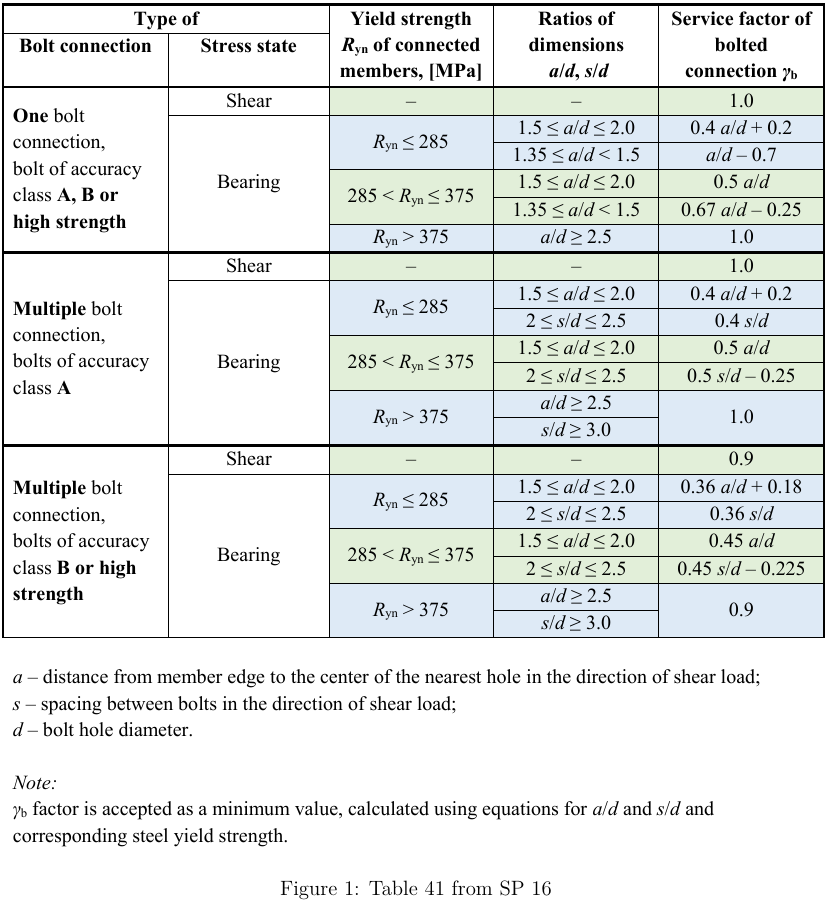A plate subject to a bearing force due to a bolt in shear is designed according to SP 16, Cl. 14.2.9 and shall satisfy:$N_s ≤ N_{bp} = R_{bp} d_b t \gamma_b \gamma_c$where: Ns – shear force in a bolt acting on a ply Nbp – bearing resistance of a ply Rbp – bearing design strength; Rbp = 1.6 · Ru for accuracy class A and Rbp = 1.35 · Ru for accuracy class B – SP 16, Table 5 Run – ultimate strength of the connected element db – bolt diameter t – thickness of the plate γb – service factor of bolt joint – SP 16, Table 41 γc – service factor – SP 16, Table 1, editable in Code setup Each plate is checked individually and the worst case is shown. SP 16 does not show the service factor of bolted connection, γb for cases outside detailing limits. Therefore, the bearing check is not performed for such cases.Friction-type connectionsFor friction-type connections, the slip is required to be limited and checked according to SP 16, Cl. 14.3. These bolts should be also checked as bearing type for ultimate limit state after slip occurs. A bolt subjected to shear force shall satisfy:$N_s \le N_{bf} = Q_{bh} \gamma_b \gamma_c$where: Ns – shear force acting on one preloaded bolt and one friction plane Nbf – shear slip resistance of one preloaded bolt and one friction plane Qbh = Rbh Abn μ / γh – design slip resistance of one preloaded bolt and one friction plane Rbh = 0.7 · Rbun – design preload in the preloaded bolt – SP 16, Cl. 6.7 Rbun – ultimate tensile resistance of the bolt Abn – tensile stress area μ – frictional coefficient for preloaded bolts – SP 16, Table 42, editable in Code setup γh – coefficient in case of bolt tightening – SP 16, Table 42 Regular holes: static loading, Δ ≤ 4 mm; dynamic loading, Δ ≤ 1 mm: γh = 1.12 for μ ≥ 0.42 γh = 1.17 for 0.35 ≤ μ < 0.42 γh = 1.30 for μ < 0.35 Oversized holes: static loading, Δ > 4 mm; dynamic loading, Δ > 1 mm: γh = 1.70 for μ < 0.35 γh = 1.35 for μ ≥ 0.35 Δ – difference between diameters of bolt hole and of bolt γb – friction joint service factor – SP 16, Cl. 14.3.4 γc – service factor – SP 16, Table 1, editable in Code setup Static or dynamic loading can be set in Code setup. Number of bolts n$$\gamma_b$$ $$n < 5$$0.8 $$5 \le n < 10$$ 0.9 $$n \ge 10$$ 1.0 Number of effective interfaces, κ, is always equal to 1, because each interface is checked separately.According to SP 16, Cl. 14.3.6, for bolts in friction-type connections loaded by combined shear and tension, friction joint service factor, γb, is multiplied by:$\gamma_b = \gamma_b \cdot \left ( 1 - \frac{N_t}{P_b} \right )$where: Nt – tensile force in a bolt Pb = Rbh Abn – preload in a bolt Rbh = 0.7 · Rbun – design preload in the preloaded bolt – SP 16, Cl. 6.7 Abn – tensile stress area Friction-type connections should be also checked for ultimate limit state. The bolt type should be changed to bearing – tension/shear interaction, the loads increased appropriately and the joint should be checked again. Check of welds according to SP It is possible to set butt welds or fillet welds and along the full edge length, partial welds or intermittent welds. Butt welds are assumed to have the same strength as the welded member and are not checked. In case of fillet welds, the weld element is inserted between interpolation links connecting plates to each other. The weld element has a specified elasto-plastic material diagram to redistribute the stress along the weld length so that the long welds, multi-orientation welds or welding to unstiffened flange have similar resistance as according to hand calculation. The most stressed weld element is decisive in the weld check.The most stressed fillet weld element of the weld is checked according to SP 16, Cl. 14.1. The length of the weld should be decreased by 10 mm according to SP 16, Cl. 14.1.16.Weld metal check:$\frac{N}{\beta_f k_f l_{we} R_{wf} \gamma_c} ≤ 1.0$Base metal check:$\frac{N}{\beta_z k_f l_{we} R_{wz} \gamma_c} ≤ 1.0$where: N – force acting on a weld element βf – coefficient for weld metal from SP 16, Table 39; coefficient is determined by Code setup – type of welding and welding position (settings of weld material) βz – coefficient for base metal from SP 16, Table 39; coefficient is determined by Code setup – type of welding and welding position (settings of weld material) kf – weld leg size, fillet weld leg ratio is assumed 1:1 $$l_{we} = \frac{l_w}{l} \cdot l_e$$ – design weld element length lw = l – 10 mm – design weld length l – actual weld length le – actual weld element length $$R_{wf} = 0.55 \frac{R_{wun}}{\gamma_{wm}}$$ – weld metal ultimate strength – SP 16, Table 4 Rwz = 0.45 Run – base metal ultimate strength – SP 16, Table 4 γc – service factor – SP 16, Table 1, editable in Code setup Rwun – standard strength of fillet weld metal from SP 16, Table D2 γwm – partial safety factor for weld metal, γwm = 1.25 for Rwun ≤ 490 MPa and γwm = 1.35 otherwise – SP 16, Table 4 Run – characteristic strength of connected steel Welding materialRwun [MPa]Rwf [MPa] E42410180 E46450200 E50490215 E60590240 E70685280 E85835340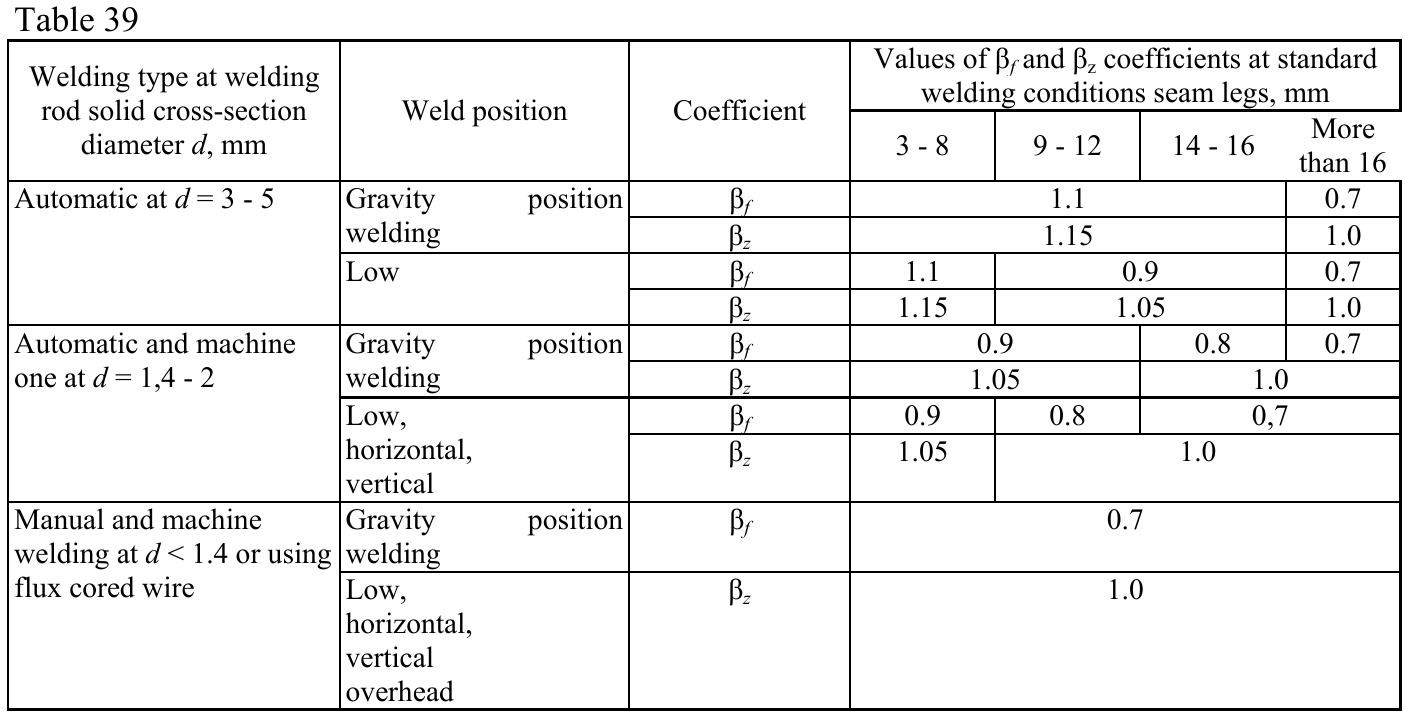The gravity type of weld position may be set at the selection of welding electrode and the welding type in the Code setup.The weld diagrams show stress according to the following formula:$\sigma = \sqrt{ \sigma_{\perp}^2 + \tau_{\perp}^2 + \tau_{\parallel}^2 }$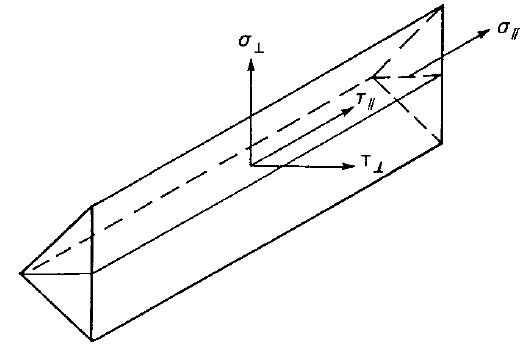Check of concrete block according to SP Concrete in compressionConcrete in compression under the base plate is checked according to SP 63.13330.2012, Cl. 8.1.44 – Calculation of reinforced concrete elements for local compression:$N \le \psi R_{b,loc} A_{b,loc}$where: N – local compressive force from an external load ψ – factor equal to 0.75 in case of non-uniform distribution of the local load across the bearing surface Rb,loc = φb Rb – design compressive resistance of concrete in case of the local impact of compressive force $$\varphi_b = 0.8 \sqrt{\frac{A_{b,max}}{A_{b,loc}}}$$ and 1.0 ≤ φb ≤ 2.5 – concentration factor taking into account the triaxial stress in concrete Rb = Rbn / γb – design value of concrete axial compressive resistance Rbn – normative axial compressive resistance of concrete γb = 1.3 – reliability factor for concrete under compression; editable in Code setup Ab,loc – application area of the compressive force (bearing area surface) determined by finite element method as area in contact between base plate and concrete block Ab,max – maximum design area established based on the following rules: gravity centres of areas Ab,loc and Ab,max coincide maximum design area is geometrically similar to application area; the slopes are 1 vertical to 2 horizontal. Transfer of shearThe shear action at the base plate is assumed to be transferred from the column to the concrete foundation by: Friction between base plate and concrete / grout Shear lug Anchor bolts AnchorsThe tensile forces in anchors include prying forces and are determined by finite element analysis.Anchors are not checked in the software. Detailing of bolts and welds and anchors according to SP Bolts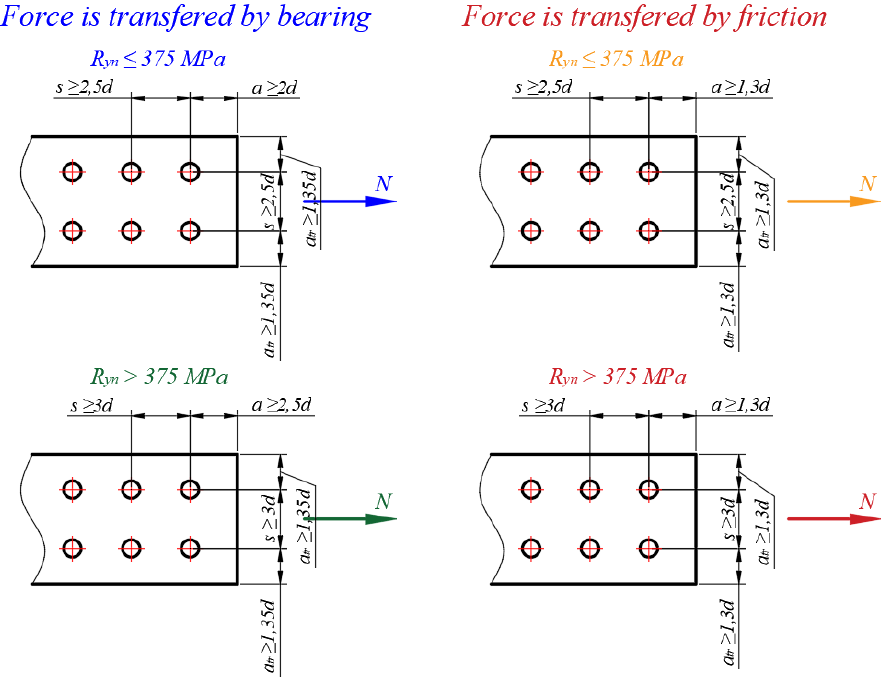Minimum pitch and minimum edge distance are checked according to SP 16, Table 40.Minimum pitch is 2.5· d for steel with Ryn ≤ 375 MPa and 3 · d otherwise.Minimum edge distance is 2 · d for steel with Ryn ≤ 375 MPa and 2.5 · d otherwise in the direction of the shear load. Minimum edge distance is 1.35 · d in the direction perpendicular to the shear load. Minimum edge distances may be smaller under some circumstances specified in SP 16, Table 40. If these conditions are met, user can deactivate the detailing check. However, the check of bolts in bearing may not be performed.Preloaded boltsMinimum pitch and minimum edge distance are checked according to SP 16, Table 40.Minimum pitch is 2.5 · d for steel with Ryn ≤ 375 MPa and 3 · d otherwise.Minimum edge distance is 1.3 · d.WeldsThe detailing of welds is checked according to SP 16, Cl. 14.1.7. The maximum fillet weld size, kf,max, should be smaller than 1.2 · tmin, where tmin is the thickness of the thinner connected plate. The minimum fillet weld size, kf,min, is checked according to SP 16, Table 38. Thickness tmax is the thickest of the plates being welded. For $$t_{min} < 0.6 \cdot t_{max}$$ – kf,min = tmin for one-sided fillet weld and $$k_{f,min} = t_{min} / \sqrt{2}$$ for two-sided fillet weld For $$t_{min} \ge 0.6 \cdot t_{max}$$ – kf,min is selected from the table below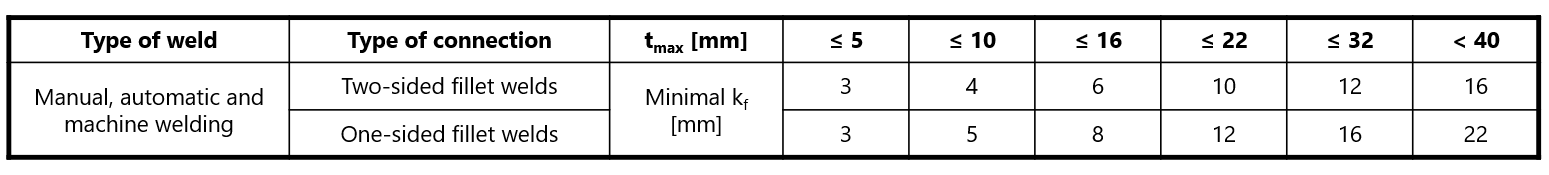Joint classification according to SP Joints are classified according to joint stiffness to: Rigid – joints with insignificant change of original angles between members, Semirigid – joints which are assumed to have the capacity to furnish a dependable and known degree of flexural restraint, Pinned – joints which do not develop bending moments. Joints are classified according to the EN 1993-1-8 – Cl. 5.2.2. Rigid – $$\frac{S_{j,ini} L_b}{E I_b} \ge k_b$$ Semirigid – $$0.5 < \frac{S_{j,ini} L_b}{E I_b} < k_b$$ Pinned – $$\frac{S_{j,ini} L_b}{E I_b} \le 0.5$$ where: Sj,ini – initial stiffness of the joint; the joint stiffness is assumed linear up to the 2/3 of Mj,Rd Lb – theoretical length of the analyzed member; set in member properties E – Young's modulus of elasticity Ib – moment of inertia of the analyzed member kb = 8 for frames where the bracing system reduces the horizontal displacement by at least 80 %; kb = 25 for other frames, provided that in every storey Kb/Kc ≥ 0.1. The value of kb = 25 is used unless the user sets "braced system" in Code setup. Mj,Rd – joint design moment resistance Kb = Ib / Lb Kc = Ic / Lc Capacity design according to SP Capacity design is using the same procedure as in EC due to missing prescriptions in Russian codes.The objective of capacity design is to confirm a building undergoes controlled ductile behaviour in order to avoid collapse in a design-level earthquake. Plastic hinge is expected to appear in dissipative item and all non-dissipative items of the joint must be able to safely transfer forces due to the yielding in the dissipative item. The dissipative item is usually a beam in moment resisting frame but it may also be e.g. an end plate. The service factor is not used for dissipative items. Two factors are assigned to the dissipative item: γov – overstrength factor – EN 1998-1, Cl. 6.2; the recommended value is γov = 1.25; editable in materials γsh – strain-hardening factor; the recommended values are γsh = 1.2 for beam in moment resisting frame, γsh = 1.0 otherwise; editable in operation The material diagram is modified according to the following figure: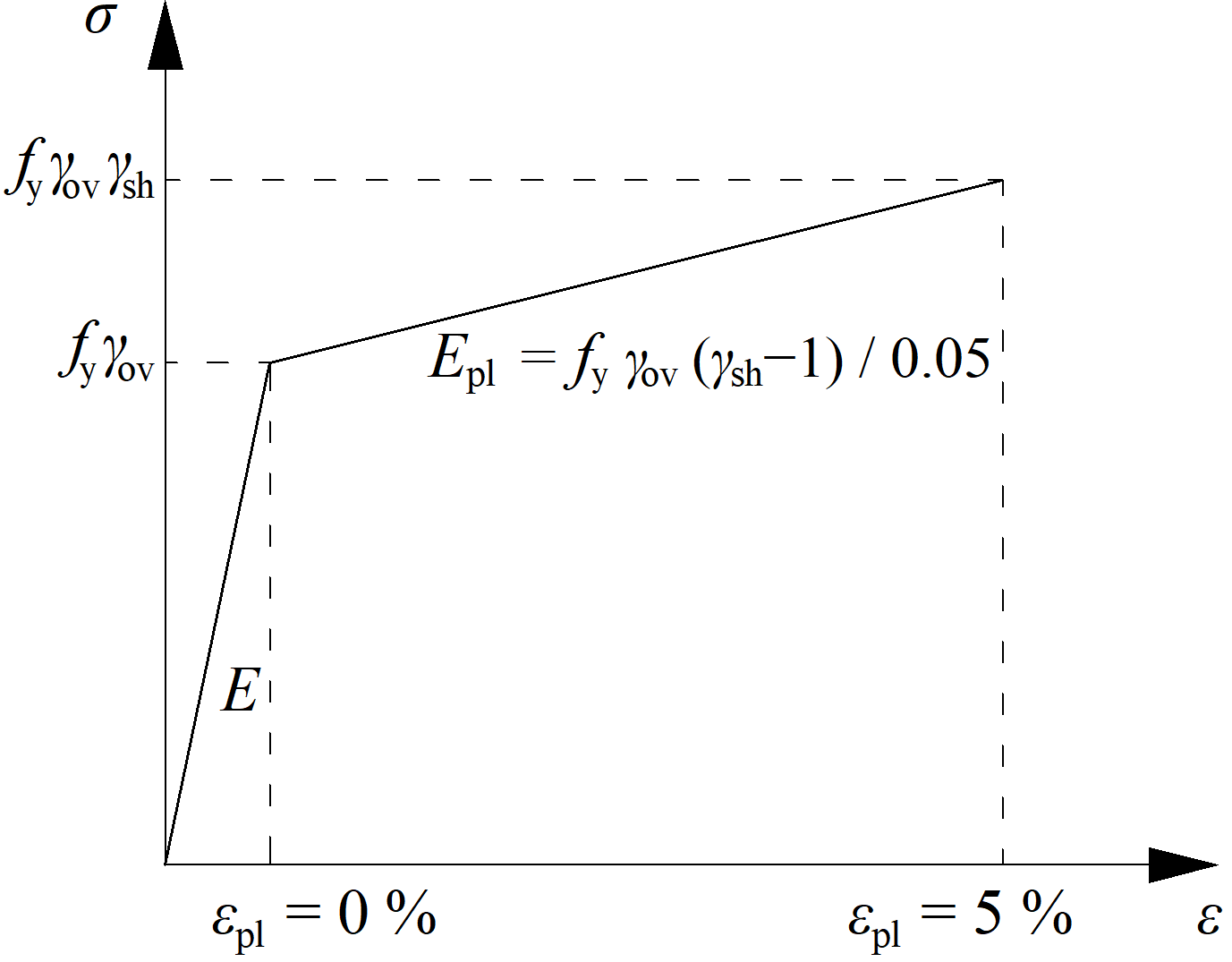The increased strength of the dissipative item allows for the input of loads that cause the plastic hinge to appear in dissipative item. In the case of moment resisting frame and beam as the dissipative item, the beam should be loaded by My,Ed = γovγshfyWpl,y and corresponding shear forceVz,Ed = –2 My,Ed / Lh, where: fy – characteristic yield strength Wpl,y – plastic section modulus Lh – distance between plastic hinges on the beam In case of asymmetric joint, the beam should be loaded by both sagging and hogging bending moments and their corresponding shear forces.The plates of dissipative items are excluded from check.$$